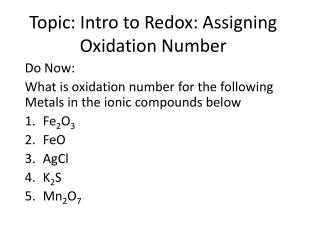DownloadDownload PresentationTopic: Intro to Redox: Assigning Oxidation Number

# Topic: Intro to Redox: Assigning Oxidation Number

Download Presentation## Topic: Intro to Redox: Assigning Oxidation Number

- - - - - - - - - - - - - - - - - - - - - - - - - - - E N D - - - - - - - - - - - - - - - - - - - - - - - - - - -
##### Presentation Transcript

1. Topic: Intro to Redox: Assigning Oxidation Number Do Now: What is oxidation number for the following Metals in the ionic compounds below Fe2O3 FeO AgCl K2S Mn2O7

2. Redox Reactions = oxidation-reduction reaction • Electrons transferred from 1 atom to another • Redox reactions: • All single-replacement rxns • All combustion rxns • Double Replace is never Redox

3. OIL RIG - memorize  O – Oxidation I - is L - loss of electron R - Reduction I - is G – gain of electron

4. REDOX Oxidation & Reduction reactions are complementary reactions • Something is oxidized - __________ electrons • While something is reduced - ________ electrons • They occur every day • Respiration • Photosynthesis • combustion loses gains

5. To understand these Redox Rxns we must first understand Oxidation numbersRules to Assigning Oxidation Numbers( oxidation # =what an atoms charge would be if it owned all it’s electron exclusively (not sharing)

6. #1: individual atom (monatomic, diatomic, polyatomic , but only 1 type of atom) = 0

7. #2for an ion oxidation number = charge Ca+2 = +2 Cl-1 = -1 Al+3 = +3

8. # 3 • When Fluorine is in a compound F is always ____________ CF4 C= ___ F = _-1_ OF2 O= ___ F = _-1_ -1 +4 +2

9. Don’t forget O2 by itself has an oxidation number of 0 # 4 • When in a Oxygen is in a compound, O is AMLOST always ________ • EXCEPT when with Fluorine • OF2O= ____ F = _-1_ • Except when O2 is bonded with group 1 or 2 metal • Then it’s a ____________________ • K2O2 = K = ____O = ____ -2 +2 Peroxide +1 -1

10. #5 H is nearly always +1 • except if bonded to metal then -1 • H2O H= ____O = ____ • H2SO4 H = ____SO4 = ____ • LiHLi = ____H = ____ • CaH2 Ca = ____H = ____ • NH3N = ____H = ____ +1 -2 +1 -2 -1 +1 +2 -1 -3 +1

11. #6 • For neutral compounds, sum of oxidation numbers = 0 • H2O 2H = +1 = +2 1O= -2 = -2 +2-2 =0 • H2SO4 2H = +1 = +2 1SO4= -2 = -2 +2-2 =0 • LiH 1Li = +1 = +1 1H= -1 = -1 +1-1 =0 • CaH2 1Ca = +2 = +2 2H= -1 = -2 +2-2 =0

12. #7 • For polyatomic ions, sum of oxidation numbers = charge of ion • SO4-21S = ___= ___ 4O= ___ = ____ ___ + ___ =-2 • NO3-11N = ___= ___ 3O= ___ = ____ ___ + ___ =-1 +6 -2 +6 +6 -8 -2 +6 -8 +5 -2 +5 +5 -2 -6 -6 +5

13. # 8 • If covalent compound – both elements are nonmetal, like to gain electrons (both negative?! – NO) • Assign negative to the more electronegative element • SiCl4: Si = +4, Cl = -1

14. K = +1, Cl = -1 Al = +3, O = -2, N = +5 Assign Oxidation #’s • KCl • CaBr2 • CO • CO2 • Al(NO3)3 • Na3PO4 • H2S • NH4+1 • SO3-2 Ca = +2, Br = -1 C = +2, O = -2 C = +4, O = -2 Na = +1, O = -2, P = +5 H = +1, S = -2 N = -3, H = +1 S = +4, O = -2